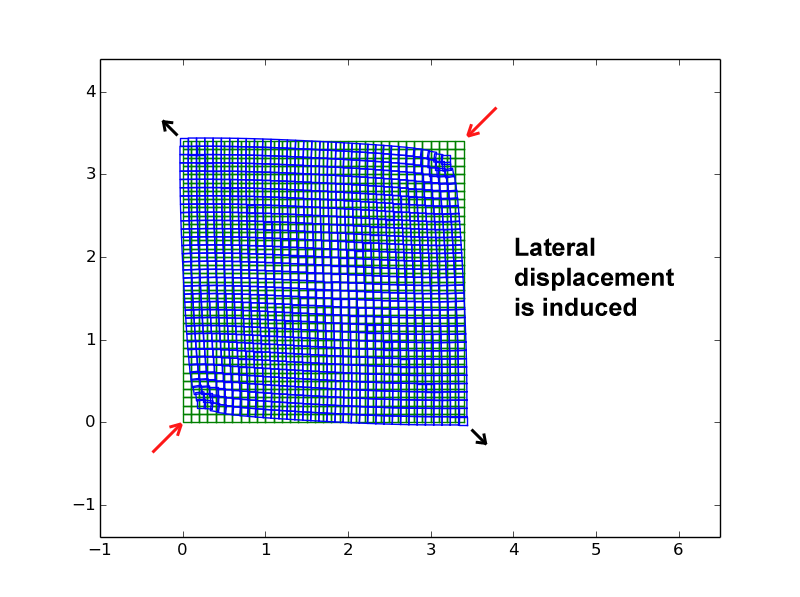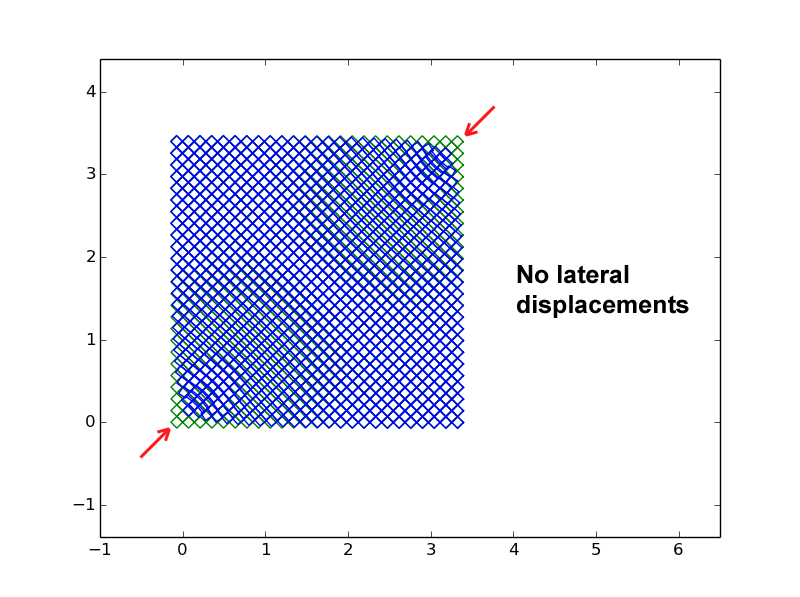## Saturday, July 19, 2014

### Intrinsic Anisotropic Poisson Effect that exists in the basic Applied Element Formulation

In my previous article, I had discussed about the AEM program for linear 2D materials that I had written in Python. Although, poisson's ratio was not considered in that program, I found that when elements are compressed (or stretched) diagonally, an intrinsic poisson effect is induced. However, such poisson effect is non existent when elements are compressed (or stretched) orthogonally. This is mainly because when the elements are compressed diagonally, the diagonal displacement induces extra force on the two lateral elements as shown in figure 1.

Note: Element properties used in all of the simulations presented here are:
Young's Modulus (E) = 207 GPa
Shear Modulus (G) = 79.1 GPa
Element Dimension (a by b) = 0.1 m by 0.1 m
Element Thickness (T) = 0.15 m
Load (L) = 1000 kN [each red arrows]
Also in all of the figures presented here, green squares represent undeformed elements and blue squares represent deformed elements. Displacements are magnified 500 times.Figure 1: Lateral displacements induced (represented by black arrows) when force (represented by red arrows) is applied in a direction diagonal to the element edges. This is the intrinsic anisotropic poisson effect induced in the basic applied element formulation.

## Rectangular Lattice

First let us look at elements arranged in a rectangular lattice. When the elements are subjected to vertical load, no lateral squeezing is found as shown in figure 2. This is as expected because no poisson formulation is applied in this basic AEM program. However, when diagonal loads are applied as shown in figure 3, we can see that the elements squeeze outwards laterally (i.e. in the perpendicular direction to the applied load).Figure 2: When elements are arranged in a rectangular lattice, vertical load causes no lateral displacements.Figure 3: When elements are arranged in rectangular lattice, diagonal loads (represented by red arrows) cause lateral displacements (represented by black arrows)

## Diagonal Lattice

Next the elements are arranged in a diagonal lattice. When it is subjected to vertical load we can see the arrangement squeezes out laterally as shown in figure 4. The poisson's ratio is approximately 0.49, which is pretty high compared to typical value of ~0.3 for steel or concrete. However, when diagonal loads are applied on the opposite corners, no lateral squeezing is found (i.e. no displacement in perpendicular direction to the applied load) as shown in figure 5. This is mainly because the load is orthogonal to the element edges.Figure 4: When elements are arranged in a diagonal lattice, vertical load causes lateral squeezing. The poisson's ratio is approximately 0.49.Figure 5: When elements are arranged in diagonal lattice, diagonal loads (represented by red arrows) cause no lateral displacements. This is mainly because the loads are orthogonal to element edges.